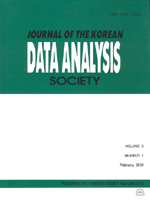상세검색
최근 검색어 전체 삭제
다국어입력
즐겨찾기0KCI등재 학술저널

# An Indirect Forced Quantitative Randomized Response Model

• 등재여부 : KCI등재
• 2013.10
• 2357 - 2365 (9 pages)

To procure a reliable information from the population related to the privacy, Warner (1965) proposed an ingenious model, called randomized response model (RRM). Since then many researchers including Eichhorn, Hayre (1983), have developed the method for the estimation of the mean of the stigmatized variable beyond the estimation of the population proportion of a sensitive attribute which was originally suggested by Warner in 1965. We suggest an indirect forced quantitative randomized response (IFQRR) model in which we use the scrambled response of Gupta et al. (2002) instead of using that of Eichhorn, Hayre (1983) which Saha (2011) used. By so doing, we can demand true response twice indirectly from respondent and we extend it to stratified population and compare the efficiency of our model with Saha (2011) model and Gupta et al. (2002) model. We can see that the suggested model is always more efficient than Saha (2011) model regardless of the values of qihj(i=1,2) under the condition of θ=1 and is more efficient than that of Gupta et al. (2002) when qihj≥q.

1. Introduction

2. Gupta et al.’s (2002) ORR model

3. An indirect forced quantitative randomized response (IFQRR) model

4. Efficiency comparison

5. Conclusions

References